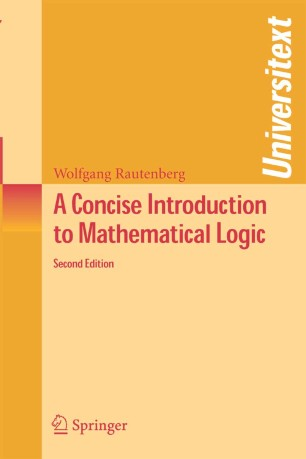# A Concise Introduction to Mathematical Logic

• Wolfgang RautenbergTextbook

Part of the Universitext book series (UTX)

1. Front Matter
Pages I-XVII
2. Pages 1-32
3. Pages 33-70
4. Pages 71-104
5. Pages 105-130
6. Pages 131-166
7. Pages 167-208
8. Pages 209-230
9. Back Matter
Pages 231-256

### Introduction

Traditional logic as a part of philosophy is one of the oldest scientific disciplines. Mathematical logic, however, is a relatively young discipline and arose from the endeavors of Peano, Frege, Russell and others to create a logistic foundation for mathematics. It steadily developed during the 20th century into a broad discipline with several sub-areas and numerous applications in mathematics, informatics, linguistics and philosophy.  While there are already several well-known textbooks on mathematical logic, this book is unique in that it is more concise than most others; the material is treated in a streamlined fashion. This allows the lecturer to select the material for a one-semester course on a topic more easily.

Although the book is intended for use as a graduate text, the first three chapters could be understood by undergraduates interested in mathematical logic.  These initial chapters cover just the material for an introductory course on mathematical logic combined with the necessary material from set theory.  Chapter 3 is partly of a descriptive nature, providing a view towards decision problems, automated theorem proving, non-standard models and related subjects.  The other chapters contain material on logic programming for computer scientists, model theory, recursion theory, Gödel's Incompleteness Theorems, and applications of mathematical logic.  Philosophical and foundational problems of mathematics are discussed where appropriate.  The author has provided exercises for each section, as well as hints or even complete solutions to most exercises at the end of the book.

From the foreword, written by Lev Beklemishev:

"…a well-written introduction to this beautiful and coherent subject. It contains classical material such as logical calculi, beginnings of model theory, and Gödel’s incompleteness theorems, as well as some topics motivated by applications, such as a chapter on logic programming.  The author has taken great care to make the exposition readable and concise."

### Keywords

Computer computer science logic mathematical logic model programming set theory

#### Authors and affiliations

• Wolfgang Rautenberg
• 1
1. 1.FB Mathematik und Informatik Inst. Mathematik IIFreie Universität BerlinBerlinGermany